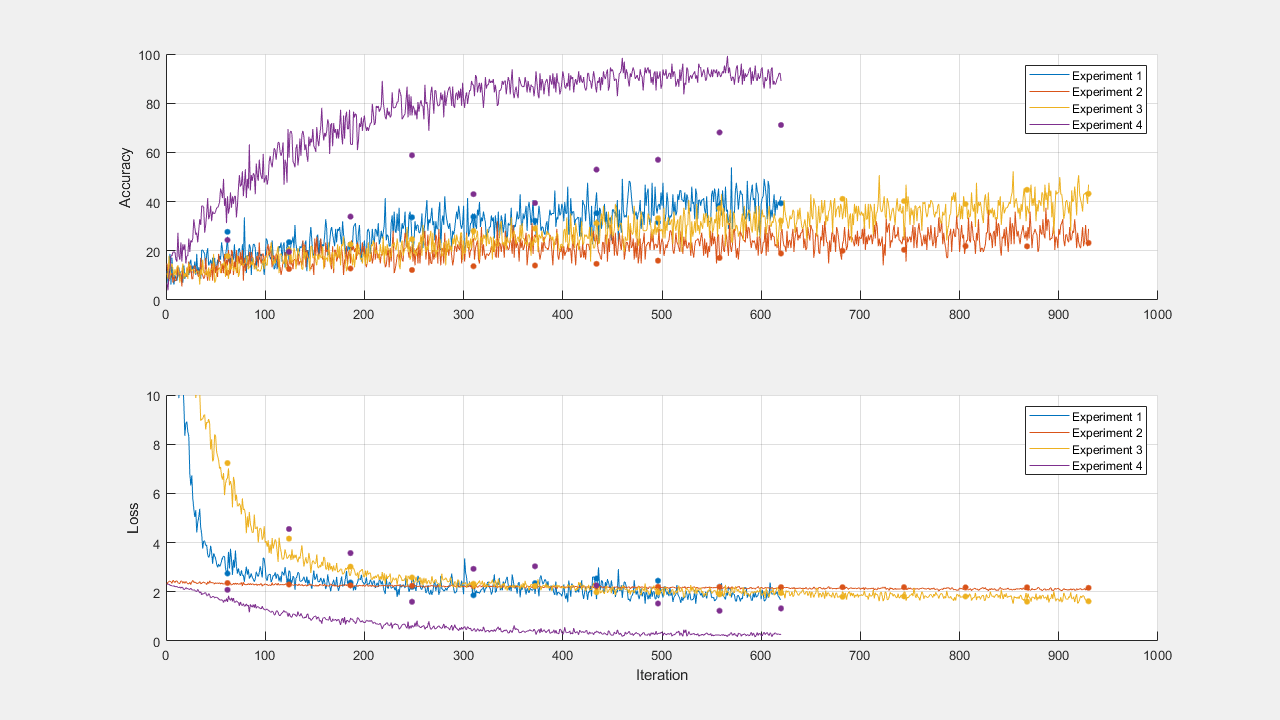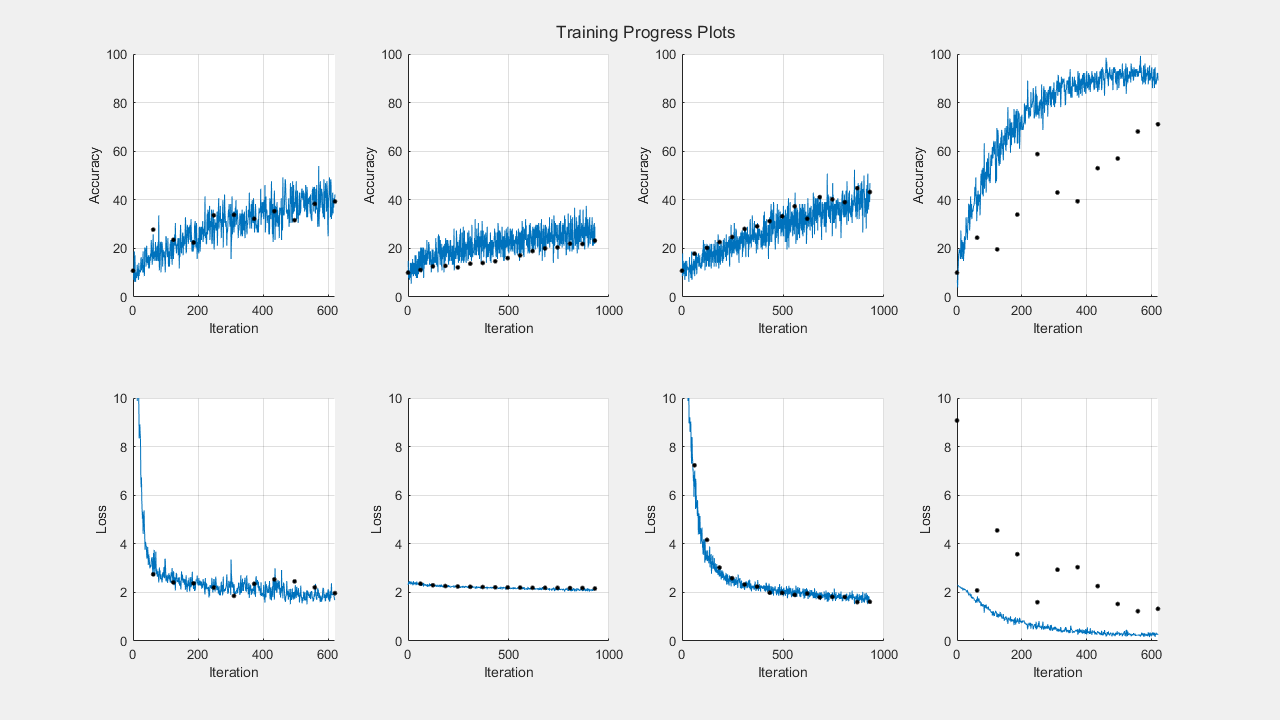Documentation

## Run Multiple Deep Learning Experiments

This example shows how to run multiple deep learning experiments on your local machine. Using this example as a template, you can modify the network layers and training options to suit your specific application needs. You can use this approach with a single or multiple GPUs. If you have a single GPU, the networks train one after the other in the background. The approach in this example enables you to continue using MATLAB® while deep learning experiments are in progress.

### Prepare Data Set

Before you can run the example, you must have access to a local copy of a deep learning data set. This example uses a data set with synthetic images of digits from 0 to 9. In the following code, change the location to point to your data set.

```datasetLocation = fullfile(matlabroot,'toolbox','nnet', ... 'nndemos','nndatasets','DigitDataset');```

If you want to run the experiments with more resources, you can run this example in a cluster in the cloud.

• Upload the data set to an Amazon S3 bucket. For an example, see Upload Deep Learning Data to the Cloud (Parallel Computing Toolbox).

• Create a cloud cluster. In MATLAB, you can create clusters in the cloud directly from the MATLAB Desktop. For more information, see Create Cloud Cluster (Parallel Computing Toolbox).

• Select your cloud cluster as the default, on the Home tab, in the Environment section, select Parallel > Select a Default Cluster.

Load the data set by using an `imageDatastore` object. Split the data set into training, validation, and test sets.

```imds = imageDatastore(datasetLocation, ... 'IncludeSubfolders',true, ... 'LabelSource','foldernames'); [imdsTrain,imdsValidation,imdsTest] = splitEachLabel(imds,0.8,0.1);```

To train the network with augmented image data, create an `augmentedImageDatastore`. Use random translations and horizontal reflections. Data augmentation helps prevent the network from overfitting and memorizing the exact details of the training images.

```imageSize = [28 28 1]; pixelRange = [-4 4]; imageAugmenter = imageDataAugmenter( ... 'RandXReflection',true, ... 'RandXTranslation',pixelRange, ... 'RandYTranslation',pixelRange); augmentedImdsTrain = augmentedImageDatastore(imageSize,imdsTrain, ... 'DataAugmentation',imageAugmenter);```

### Train Networks in Parallel

Start a parallel pool with as many workers as GPUs. You can check the number of available GPUs by using the `gpuDeviceCount` function. MATLAB assigns a different GPU to each worker. By default, `parpool` uses your default cluster profile. If you have not changed the default, it is `local`. This example was run using a machine with 2 GPUs.

```numGPUs = 2; parpool(numGPUs);```
```Starting parallel pool (parpool) using the 'local' profile ... Connected to the parallel pool (number of workers: 2). ```

To send training progress information from the workers during training, use a `parallel.pool.DataQueue` object. To learn more about how to use data queues to obtain feedback during training, see the example Use parfeval to Train Multiple Deep Learning Networks (Parallel Computing Toolbox).

`dataqueue = parallel.pool.DataQueue;`

Define the network layers and training options. For code readability, you can define them in a separate function that returns several network architectures and training options. In this case, `networkLayersAndOptions` returns a cell array of network layers and an array of training options of the same length. Open this example in MATLAB and then click `networkLayersAndOptions` to open the supporting function `networkLayersAndOptions`. Paste in your own network layers and options. The file contains sample training options that show how to send information to the data queue using an output function.

`[layersCell,options] = networkLayersAndOptions(augmentedImdsTrain,imdsValidation,dataqueue);`

Prepare the training progress plots, and set a callback function to update these plots after each worker sends data to the queue. `preparePlots` and `updatePlots` are supporting functions for this example.

`handles = preparePlots(numel(layersCell));``afterEach(dataqueue,@(data) updatePlots(handles,data));`

To hold the computation results in parallel workers, use future objects. Preallocate an array of future objects for the result of each training.

`trainingFuture(1:numel(layersCell)) = parallel.FevalFuture;`

Loop through the network layers and options by using a `for` loop, and use `parfeval` to train the networks on a parallel worker. To request two output arguments from `trainNetwork`, specify `2` as the second input argument to `parfeval`.

```for i=1:numel(layersCell) trainingFuture(i) = parfeval(@trainNetwork,2,augmentedImdsTrain,layersCell{i},options(i)); end```

`parfeval` does not block MATLAB, so you can continue working while the computations take place.

To fetch results from future objects, use the `fetchOutputs` function. For this example, fetch the trained networks and their training information. `fetchOutputs` blocks MATLAB until the results are available. This step can take a few minutes.

`[network,trainingInfo] = fetchOutputs(trainingFuture);`Save the results to disk using the `save` function. To load the results again later, use the `load` function.

`save(['experiment-' datestr(now,'yyyymmddTHHMMSS')],'network','trainingInfo');`

### Plot Results

After the networks complete training, plot their training progress by using the information in `trainingInfo`.

Use subplots to distribute the different plots for each network. For this example, use the first row of subplots to plot the training accuracy against the number of epoch along with the validation accuracy.

```figure('Units','normalized','Position',[0.1 0.1 0.6 0.6]); title('Training Progress Plots'); for i=1:numel(layersCell) subplot(2,numel(layersCell),i); hold on; grid on; ylim([0 100]); iterationsPerEpoch = floor(augmentedImdsTrain.NumObservations/options(i).MiniBatchSize); epoch = (1:numel(trainingInfo(i).TrainingAccuracy))/iterationsPerEpoch; plot(epoch,trainingInfo(i).TrainingAccuracy); plot(epoch,trainingInfo(i).ValidationAccuracy,'.k','MarkerSize',10); end subplot(2,numel(layersCell),1), ylabel('Accuracy');```

Then, use the second row of subplots to plot the training loss against the number of epoch along with the validation loss.

```for i=1:numel(layersCell) subplot(2,numel(layersCell),numel(layersCell) + i); hold on; grid on; ylim([0 max([trainingInfo.TrainingLoss])]); iterationsPerEpoch = floor(augmentedImdsTrain.NumObservations/options(i).MiniBatchSize); epoch = (1:numel(trainingInfo(i).TrainingAccuracy))/iterationsPerEpoch; plot(epoch,trainingInfo(i).TrainingLoss); plot(epoch,trainingInfo(i).ValidationLoss,'.k','MarkerSize',10); xlabel('Epoch'); end subplot(2,numel(layersCell),numel(layersCell)+1), ylabel('Loss');```After you choose a network, you can use `classify` and obtain its accuracy on the test data `imdsTest`.